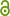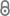On polynomially many queries to NP or QMA oracles

S. Gharibian, D. Rudolph, To Appear in 13th Innovations in Theoretical Computer Science (ITCS 2022) (2021).https://arxiv.org/abs/2111.02296
Preprint | English
Author
Abstract
We study the complexity of problems solvable in deterministic polynomial time with access to an NP or Quantum Merlin-Arthur (QMA)-oracle, such as $P^{NP}$ and $P^{QMA}$, respectively. The former allows one to classify problems more finely than the Polynomial-Time Hierarchy (PH), whereas the latter characterizes physically motivated problems such as Approximate Simulation (APX-SIM) [Ambainis, CCC 2014]. In this area, a central role has been played by the classes $P^{NP[\log]}$ and $P^{QMA[\log]}$, defined identically to $P^{NP}$ and $P^{QMA}$, except that only logarithmically many oracle queries are allowed. Here, [Gottlob, FOCS 1993] showed that if the adaptive queries made by a $P^{NP}$ machine have a "query graph" which is a tree, then this computation can be simulated in $P^{NP[\log]}$. In this work, we first show that for any verification class $C\in\{NP,MA,QCMA,QMA,QMA(2),NEXP,QMA_{\exp}\}$, any $P^C$ machine with a query graph of "separator number" $s$ can be simulated using deterministic time $\exp(s\log n)$ and $s\log n$ queries to a $C$-oracle. When $s\in O(1)$ (which includes the case of $O(1)$-treewidth, and thus also of trees), this gives an upper bound of $P^{C[\log]}$, and when $s\in O(\log^k(n))$, this yields bound $QP^{C[\log^{k+1}]}$ (QP meaning quasi-polynomial time). We next show how to combine Gottlob's "admissible-weighting function" framework with the "flag-qubit" framework of [Watson, Bausch, Gharibian, 2020], obtaining a unified approach for embedding $P^C$ computations directly into APX-SIM instances in a black-box fashion. Finally, we formalize a simple no-go statement about polynomials (c.f. [Krentel, STOC 1986]): Given a multi-linear polynomial $p$ specified via an arithmetic circuit, if one can "weakly compress" $p$ so that its optimal value requires $m$ bits to represent, then $P^{NP}$ can be decided with only $m$ queries to an NP-oracle.
Publishing Year
Journal Title
To appear in 13th Innovations in Theoretical Computer Science (ITCS 2022)
LibreCat-ID

Cite this

Gharibian S, Rudolph D. On polynomially many queries to NP or QMA oracles. To appear in 13th Innovations in Theoretical Computer Science (ITCS 2022). Published online 2021.
Gharibian, S., & Rudolph, D. (2021). On polynomially many queries to NP or QMA oracles. In To appear in 13th Innovations in Theoretical Computer Science (ITCS 2022).
@article{Gharibian_Rudolph_2021, title={On polynomially many queries to NP or QMA oracles}, journal={To appear in 13th Innovations in Theoretical Computer Science (ITCS 2022)}, author={Gharibian, Sevag and Rudolph, Dorian}, year={2021} }
Gharibian, Sevag, and Dorian Rudolph. “On Polynomially Many Queries to NP or QMA Oracles.” To Appear in 13th Innovations in Theoretical Computer Science (ITCS 2022), 2021.
S. Gharibian and D. Rudolph, “On polynomially many queries to NP or QMA oracles,” To appear in 13th Innovations in Theoretical Computer Science (ITCS 2022). 2021.
Gharibian, Sevag, and Dorian Rudolph. “On Polynomially Many Queries to NP or QMA Oracles.” To Appear in 13th Innovations in Theoretical Computer Science (ITCS 2022), 2021.
All files available under the following license(s):Closed Access